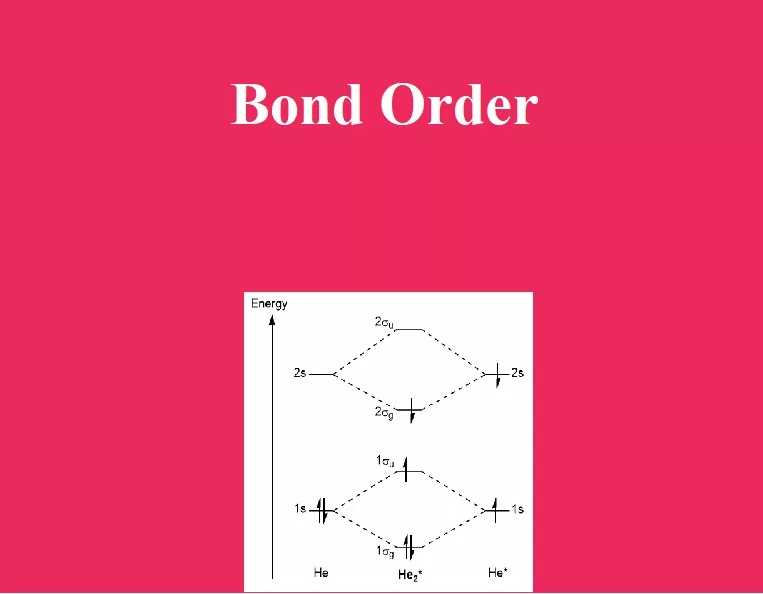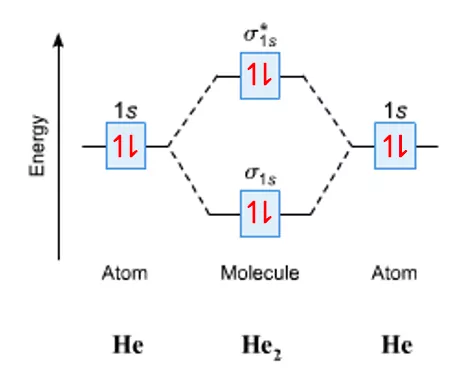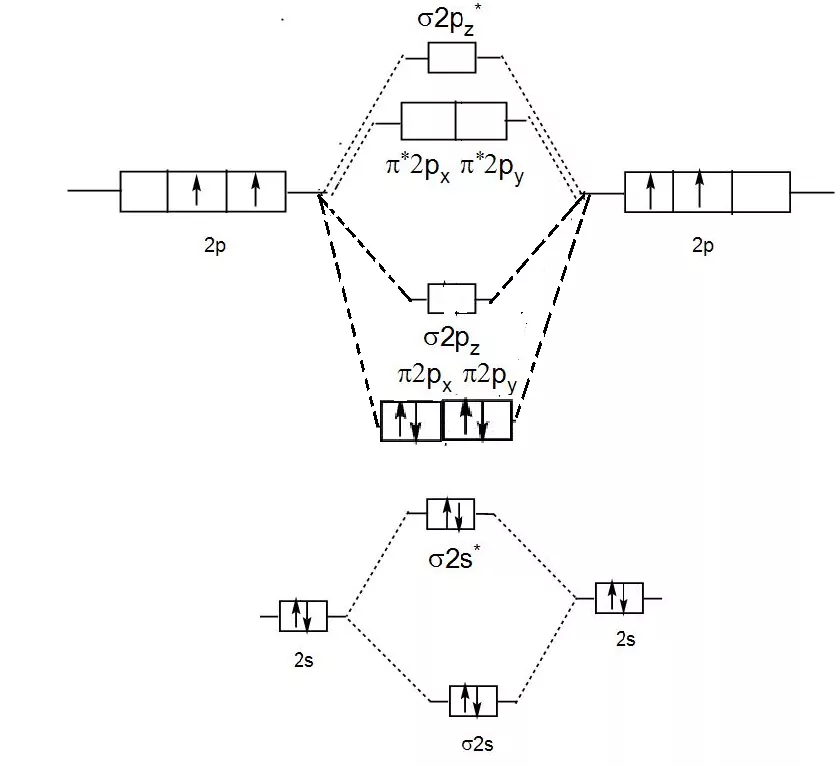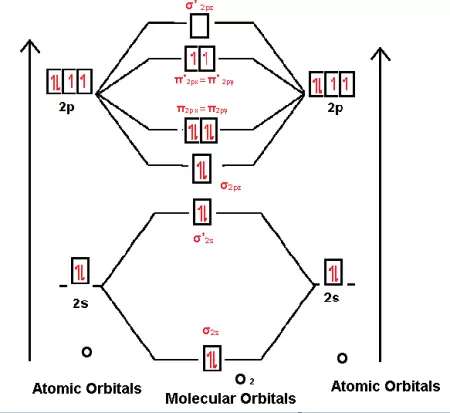# Bond order# Bond Order

It was introduced by Linus Pauling. Bond order is defined as half the difference between the number of bonding electrons and antibonding electrons.
In a covalent bond between two atoms, a single bond has a bond order of one, a double bond has a bond order of two, a triple bond has a bond order of three, and so on. The bond number gives an indication of the stability of a bond. Isoelectronic species have the same bond number.

## Bond Order Formula:

The Bond Order Formula can be defined as half of the difference between the number of electrons in bonding orbitals and antibonding orbitals.
B.O = ½ [Nb – Na]
Where,
Nb is the number of bonding electrons
Na is the number of antibonding electrons
If the bond order is zero, the molecule cannot form. The higher bond orders indicate greater stability for the new molecule. In molecules that have resonance, the B.O. does not need to be an integer.

Bond Order in Molecular Orbital Theory:
Let us take few examples to find bond order using the Molecular orbital energy level diagram and configuration.
1. Dihydrogen (H2): One hydrogen atom contains 1 electron, as we have two hydrogen atoms in dihydrogen so we have to write a configuration for two electrons.
Configuration: (σ1s)2
Molecular Orbital Energy Level Diagram:

B.O. = ½ [Bonding Electrons – Antibonding electrons]
As we have 2 electrons in bonding only
Therefore B.O. = ½ [2 – 0] = 1

2. Helium (He2): One He atom contains 2 electrons, as we have two He atoms in He2 so we have to write configuration for 4 electrons (i.e 2 + 2).
Configuration of He2 = (σ1s)2  (σ*1s)2
Molecular Orbital Energy Level Diagram:B.O. = ½ [Bonding Electrons – Antibonding electrons]
As we have 2 electrons in bonding and two electrons in antibonding
Therefore B.O. = ½ [2 – 2] = 0
As B.O is zero the He2 molecule does not exist

3. Carbon (C2): One Carbon atom contains 6 electrons, as we have two carbon atoms in C2 so we have to write a configuration for 12 electrons (i.e 6 + 6).
Configuration of C2 = (σ1s)2  (σ*1s)2  (σ2s)2  (σ*2s)2   [л(2px)2 = л(2py)2]
Molecular Orbital Energy Level Diagram:B.O. = ½ [Bonding Electrons – Antibonding electrons]
As we have 8 electrons in bonding and 4 electrons in antibonding
Therefore B.O. = ½ [8 – 4] = 2

4. Oxygen (O2): One Oxygen atom contains 8 electrons, as we have two Oxygen atoms in O2 so we have to write a configuration for 16 electrons (i.e 8 + 8).
Configuration of O2 = (σ1s)2  (σ*1s)2  (σ2s)2  (σ*2s)2  (σ2s)2  [л(2px)2 = л(2py)2]
*(2px)1 = л *(2py)1]
Molecular Orbital Energy Level Diagram:B.O.= ½ [Bonding Electrons – Antibonding electrons]
As we have 10 electrons in bonding and 6 electrons in antibonding
Therefore B.O. = ½ [10 – 6] = 2